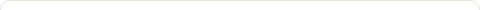# Einstein speaks on General Relativity

Posted on: November 22, 2009

Einstein speaks on General Relativity; (Nov. 20, 2009)

I have already posted two articles in the series “Einstein speaks on…” This article describes Einstein’s theory of restricted relativity and then his concept for General Relativity. It is a theory meant to extend physics of fields (for example electrical and magnetic fields among others) to all natural phenomena, including gravity. Einstein declares that there was nothing speculative in his theory but it was adapted to observed facts.

The fundamentals are that the speed of light is constant in the void and that all systems of inertia are equally valid (each system of inertia has its own metric time). The experience of Michelson has demonstrated these fundamentals. The theory of restrained relativity adopts the continuum of space coordinates and time as absolute since they are measured by clocks and rigid bodies with a twist: the coordinates become relative because they depend on the movement of the selected system of inertia.

The theory of General Relativity is based on the verified numerical correspondence of inertia mass and weight. This discovery is obtained when coordinates posses relative accelerations with one another; thus each system of inertia has its own field of gravitation. Consequently, the movement of solid bodies does not correspond to the Euclid geometry as well as the movement of clocks. The coordinates of space-time are no longer independent. This new kind of metrics existed mathematically thanks to the works of Gauss and Riemann.

Ernst Mach realized that classical mechanics movement is described without reference to the causes; thus, there are no movements but those in relation to other movements.  In this case, acceleration in classical mechanics can no longer be conceived with relative movement; Newton had to imagine a physical space where acceleration would exist and he logically announced an absolute space that did not satisfy Newton but that worked for two centuries. Mach tried to modify the equations so that they could be used in reference to a space represented by the other bodies under study.  Mach’s attempts failed in regard of the scientific knowledge of his time.

We know that space is influenced by the surrounding bodies and so far, I cannot think the general Relativity may surmount satisfactorily this difficulty except by considering space as a closed universe, assuming that the average density of matters in the universe has a finite value, however small it might be.

### 1 Response to "Einstein speaks on General Relativity"I know the bending of light lead a phenomenon of gravitation lensing, where multiple images of the same distances . gravity has the affect how planets rotate on axes. and how flying saucers react on free gravitational energy .. which its called that form of energy when positive and negative energy is used. many factors what we seek into space exploration.### Blog Stats

• 1,494,721 hits